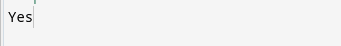# Check if there are at least T continuous blocks of 0s or not in a Binary Matrix

## Question:

You are given a binary matrix Mat (with values 0 or 1) with N rows and M columns. The task is to print “Yes” if both the below-given conditions are satisfied:

1. There are at-least 2*max(N, M) cells with value 0s.
2. There exist at least T continuous blocks of 0s where T is the GCD(greatest common divisor) of N and M. A continuous block is described as a continuous stretch from row to row, column to column, or diagonally.

Otherwise, print “No.”

Input:

First Line: Number of rows N.

Second Line: Number of columns M.

Third Line: A binary Matrix Mat.

Output:

Print “Yes” or “No.”

The questions you should ask the interviewer:

1. What are the constraints on n and m?

Examples:

Alert Ninjas:  Don’t stop now! Enroll in the Competitive Programming course today and start coding. Learn the art of writing time and space-efficient code and compete in code competitions worldwide.

Recommended: Please try to solve it yourself before moving on to the solution.

## Solution:

### Idea:

1. The idea is to count the number of cells with 0. If it satisfies condition 1, then move forward; otherwise, return No.
2. Find the gcd of M and N and use a depth-first search to find the number of connected components with a value of 0.

### Algorithm:

1. Initialize a variable zero and, using for loops, count the number of 0s in the matrix. If zero <  2*max(N, M), return “No” to the function.
2. Maintain a count variable to track the number of continuous blocks of 0s.
3. Using two for loops iterate over the matrix, for(int i = 0 ; i < N; i++) { for (int j = 0 ; j < M; j++) {, for every Mat[i][j] which is 0, increase the count and apply DFS to cover all the cells of that block.
4. If value of count >= gcd(N,M) , return “Yes”.
5. Otherwise, return “No” in the end.

C++

Output:## FAQs

1. What is a binary Matrix?
In a binary matrix, a cell can have only two values: 0 or 1.

2. How to solve the “Find the number of Islands” matrix problem?
Practice and solve here.

3. Where can I find important mathematical concepts used in coding?
You can learn the important mathematical concepts asked in the interviews from here.

## Key Takeaways

This article solved one of the important interview questions: Check if there are T continuous blocks of 0s or not in a Binary Matrix of size N*M.

Here we used DFS to solve the problem in O(N*M) time complexity.

Learn and Practice DFS from here.

Apart from that, you can use CodeStudio to practice a wide range of DSA questions asked in lots of interviews. It will assist you in mastering efficient coding techniques, and you will also get interview experiences from people working in big companies.